# 算法-数组：移除元素<font size="4">我们都知道数据结构和算法很重要，而且在校招和社招中，是必考的点，很多公司一上来就是手撕算法题，由此可以看出算法的重要性。我之前也做过一些算法题目，但是没有系统总结，而且没有坚持下来，总是断断续续的，希望从现在开始能养成习惯，每天坚持做题。我是决定跟着卡哥的路线来刷题，中间如果遇到一些不熟悉的数据结构的话，我还会去补补基础。</font>

## 思路

• 快指针：寻找新数组元素，新数组就是不含有目标元素的数组
• 慢指针：指向更新新数组下标的位置 代码
``````class Solution {
public int removeElement(int[] nums, int val) {
//快慢指针
int slow=0;
int fast;
for (fast=0;fast<nums.length;fast++){
if (nums[fast]==val){
continue;
}else{
nums[slow]=nums[fast];
slow++;
}
}
return slow;

}
}``````

## 图解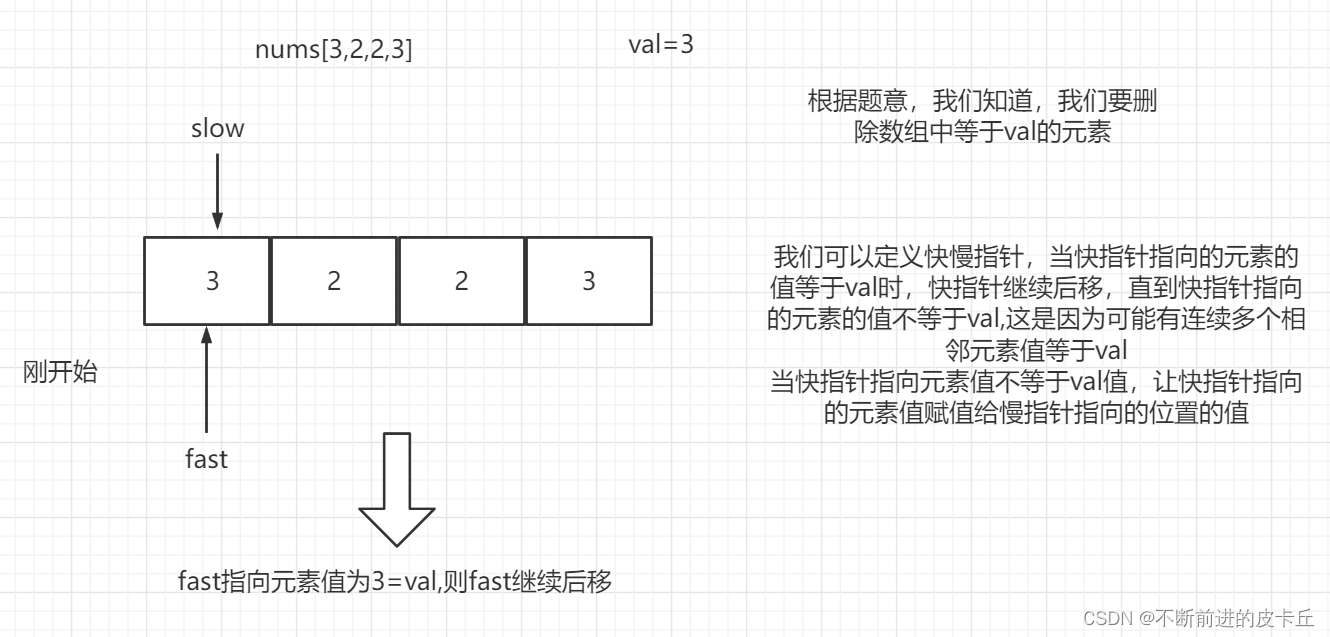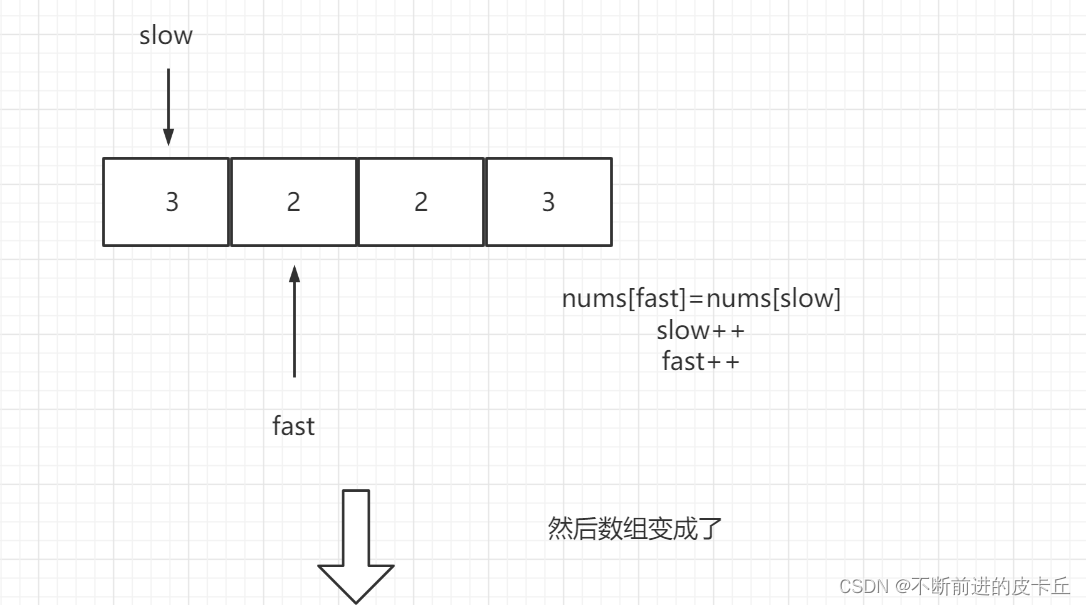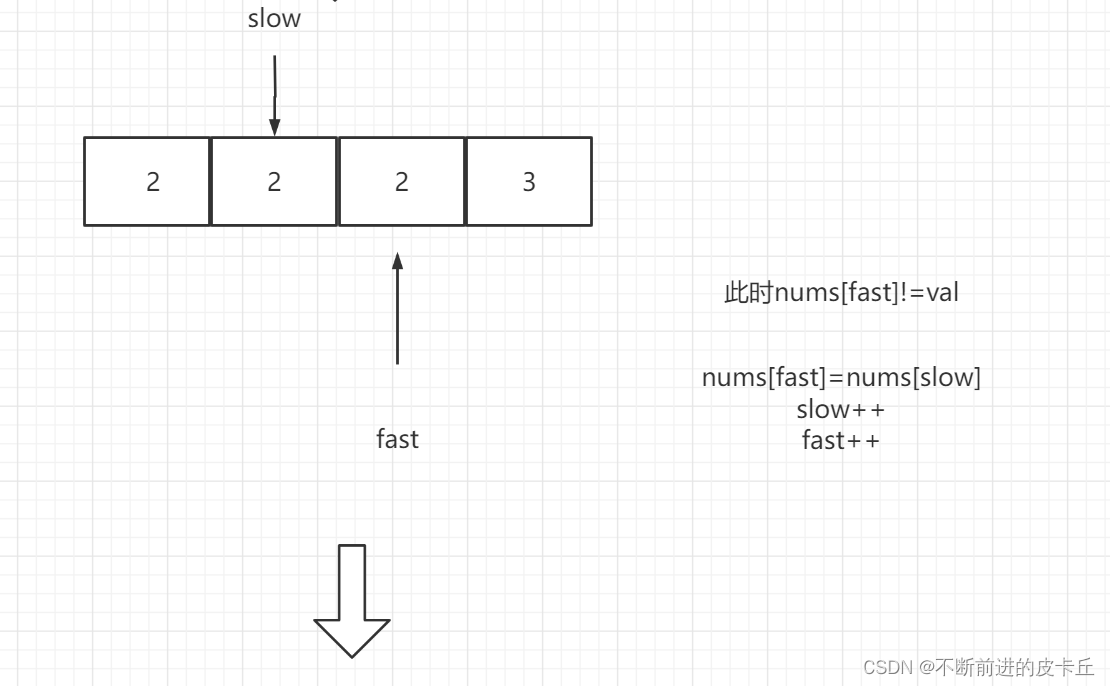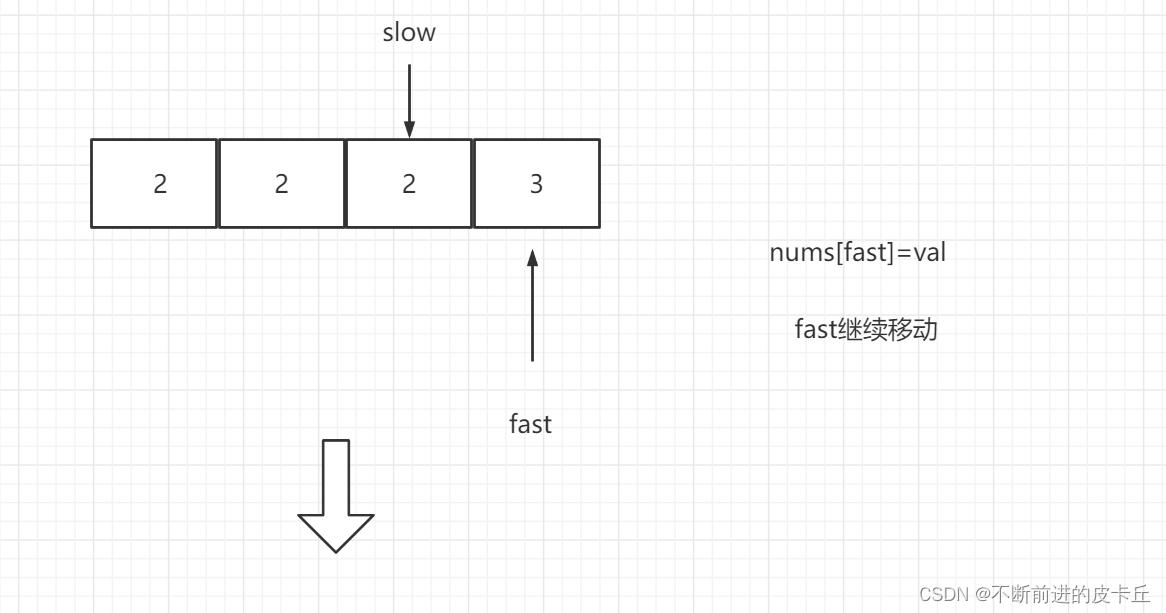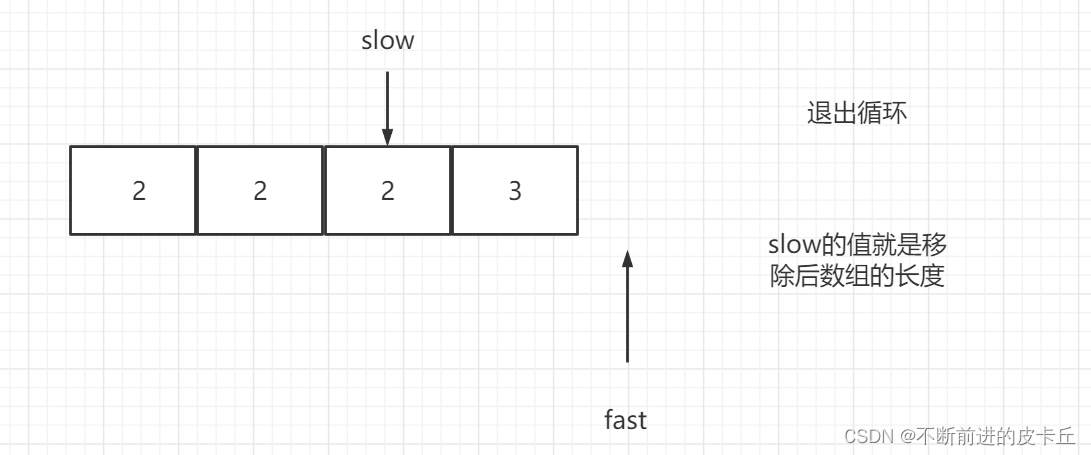## 26.删除排序数组中的重复项

1 <= nums.length <= 3 * 104 -104 <= nums[i] <= 104 nums 已按 升序 排列

### 思路

• 题目已知是升序
• 使用快慢指针，如果有重复，肯定是和相邻元素比较
• 当快指针指向元素和慢指针指向元素相等时，就让快指针后移，因为可能存在连续多个重复的元素
• 当快指针指向元素和慢指针指向元素不同时，就让慢指针后移，然后让快指针指向的元素值赋值给慢指针指向位置

### 代码

``````class Solution {
public int removeDuplicates(int[] nums) {
//快慢指针，如果有重复，肯定是和相邻元素比较
int slow=0;
int fast;
for (fast=1;fast<nums.length;fast++){
if (nums[fast]!=nums[slow]){
nums[++slow]=nums[fast];
}
}

return slow+1;
}
}``````

## 977有序数组的平方

977.有序数组的平方 给你一个按 非递减顺序 排序的整数数组 nums，返回 每个数字的平方 组成的新数组，要求也按 非递减顺序 排序。 示例 1：

1 <= nums.length <= 104 -104 <= nums[i] <= 104 nums 已按 非递减顺序 排序

### 思路

• 数组是递增的
• 因为符号的原因所以，我们不能确定它们平方后绝对值的大小关系，不过，我们知道对于负数来说，如果它的平方比较大，那么它应该排在前面，如果是整数则排在后面
• 我们通过双指针，一个指向头，一个指向尾，然后额外使用一个数组空间即可

### 代码

``````class Solution {
public int[] sortedSquares(int[] nums) {
/** * 递增排列 * 前面的负数中绝对值大的 * 后面的是正数中绝对值大的 * 对它们进行比较就可以了 * 双指针 */
int left=0;
int right=nums.length-1;
int[]ans=new int[nums.length];
int index=right;
while (left<=right){
if (Math.abs(nums[left])<Math.abs(nums[right])){
//说明左边的绝对值小
ans[index]=nums[right]*nums[right];
right--;
}else{
//说明左边大
ans[index]=nums[left]*nums[left];
left++;
}
index--;
}
return ans;
}
}``````

## 844比较含退格的字符串

### 思路

• 如果扫描到#则要把前一个字符覆盖
• 使用快慢指针
• 如果快指针指向的是#，慢指针指向的不是0，则慢指针前移
• 如果快指针指向的不是#,则把快指针指向的字符覆盖慢指针指向的字符

### 代码

``````class Solution {
public  boolean backspaceCompare(String s,String t){

return message(s).equals(message(t));
}
/** * 写一个方法来看看最终的文本信息 */
public  String message(String str){
char[] chars = str.toCharArray();

//如果扫描到#则要把前面一个覆盖
//快慢指针
//如果快指针指向的是#，则继续后移，直到找到一个非#
//如果快指针指向的不是#,则把快指针指向的字符覆盖慢指针指向的字符
int slow=0;
int fast;
for (fast=0;fast<chars.length;fast++){
if (chars[fast]!='#'){

chars[ slow++]=chars[fast];

}else if (chars[fast]=='#'&& slow!=0){
slow--;
}
}
//返回字符串
return String.valueOf(chars,0,slow);
}
}``````

-- 展开阅读全文 --

« 上一篇 07-24